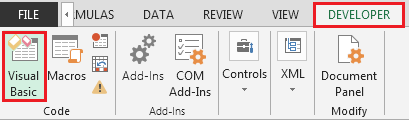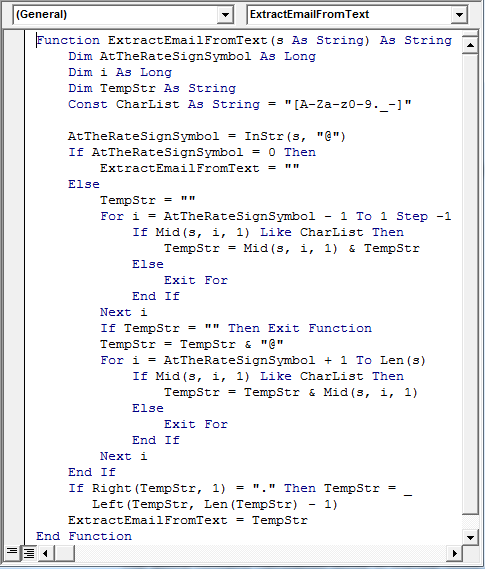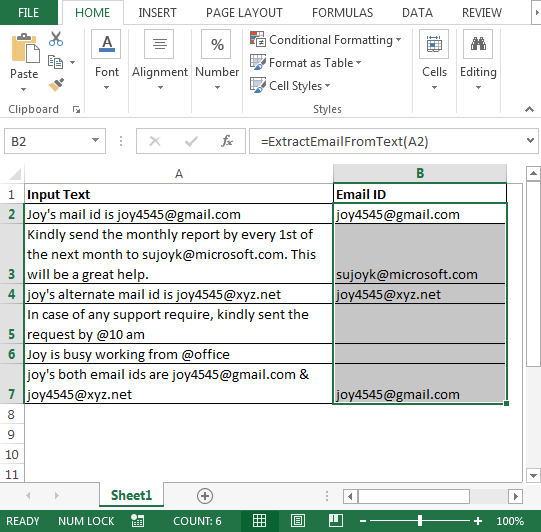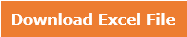# UDF - Extracting Email Address from Text

Question): The data I have contains too much information in text format. I want a VBA code to help me to extract as many email ids from the text to minimize my manual efforts.

We need to follow the below steps:

• Click on Developer tab
• From Code group, select Visual BasicEnter the following code in the standard module:

Function ExtractEmailFromText(s As String) As String    Dim AtTheRateSignSymbol As Long    Dim i As Long

Dim TempStr As String

Const CharList As String = "[A-Za-z0-9._-]"

AtTheRateSignSymbol = InStr(s, "@")

If AtTheRateSignSymbol = 0 Then

ExtractEmailFromText = ""

Else

TempStr = ""

For i = AtTheRateSignSymbol - 1 To 1 Step -1

If Mid(s, i, 1) Like CharList Then

TempStr = Mid(s, i, 1) & TempStr

Else

Exit For

End If

Next i

If TempStr = "" Then Exit Function

TempStr = TempStr & "@"

For i = AtTheRateSignSymbol + 1 To Len(s)

If Mid(s, i, 1) Like CharList Then

TempStr = TempStr & Mid(s, i, 1)

Else

Exit For

End If

Next i

End If

If Right(TempStr, 1) = "." Then TempStr = _

Left(TempStr, Len(TempStr) - 1)

ExtractEmailFromText = TempStr

End Function• In cell B2, the formula is
• =ExtractEmailFromText(A2)

We will get the result. Refer below snapshot:The above code will extract first email address in case there is more than 1 email id in a cell.

In this way, we can retrieve email is from text.